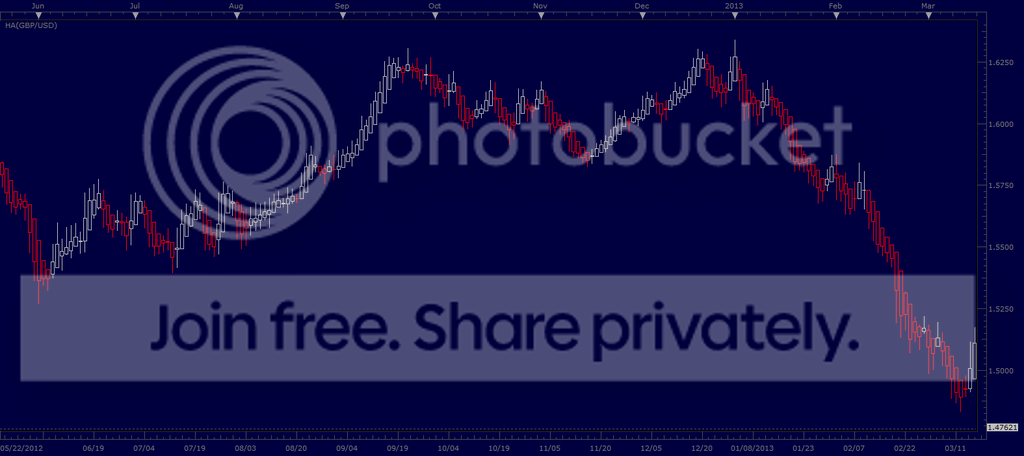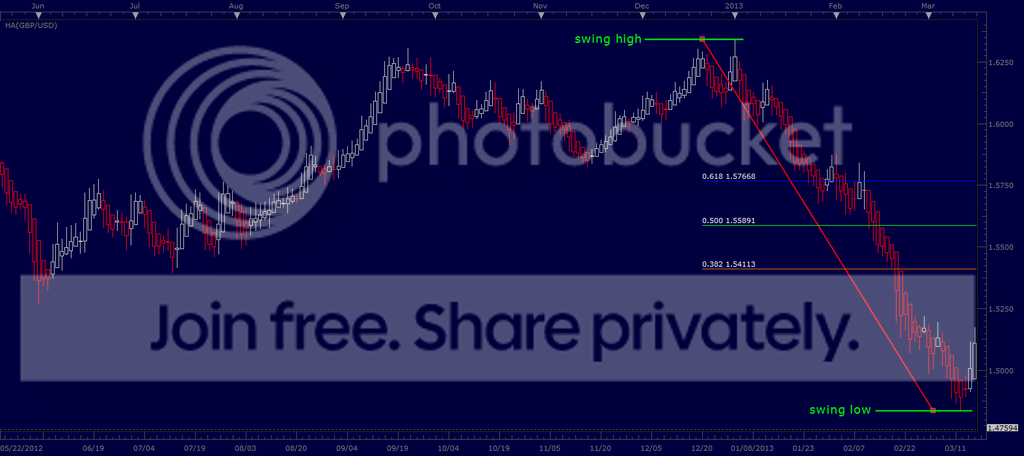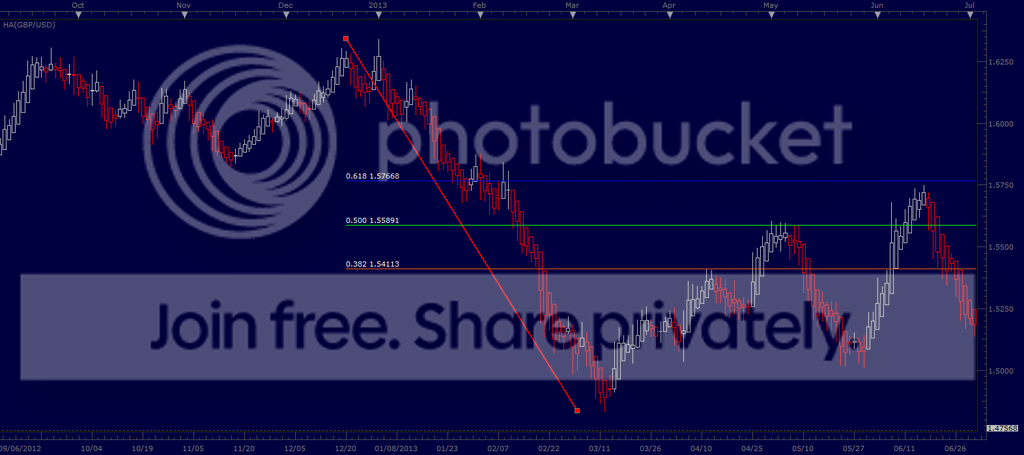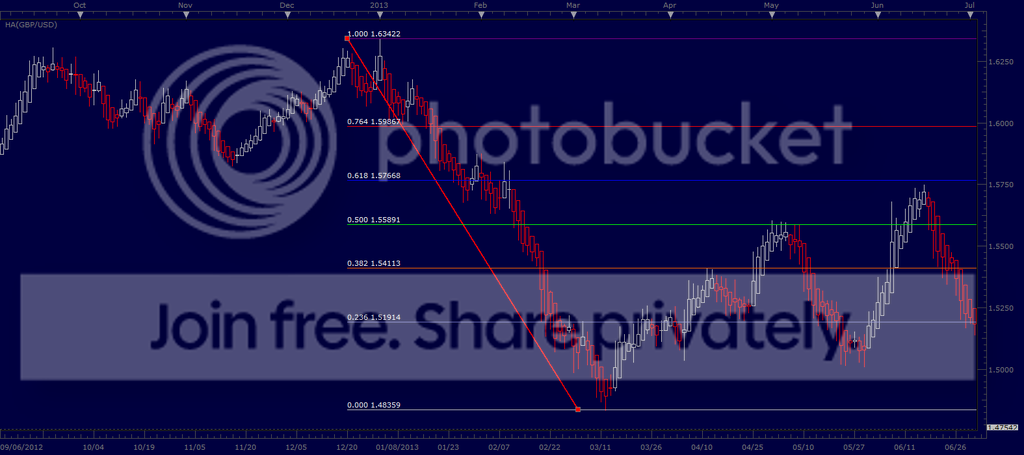# Fib retracement

What do the percents on the fib retracements mean?

Let’s use an example.

Let’s say it’s March 15, 2013, and you’re looking at a GBP/USD Daily chart.A large swing to the downside has been unfolding for a couple of months (from January to March). You think that this prolonged down-move is over-extended, and you’re anticipating a retracement.

[B]The Fibonacci retracement tool simply projects possible retracement levels as percentages of the down-move.[/B] So, let’s drop a Fibonacci retracement on the January-March price swing.We could have included several additional levels (percentages) in our Fib, but these three — 38.2%, 50%, and 61.8% are the most commonly used.

Let’s scroll forward in time, and see whether GBP/USD retraced a portion of its January-March down-move, and if so how the actual retracement compares to the Fibonacci projected retracements of 38.2%, 50%, and 61.8%.Finally, let’s put some additional percentages, between 0% and 100%, on our Fib, to make sure you can visualize how the Fibonacci retracement tool divides [B]a downward price swing[/B] into percentages of the high-low price range.If there were no retracement, at all, then obviously price would not rise above the 0% level (meaning zero retracement).

On the other hand, if price retraced all of the January-March down-move, then it would rise to (or above) the 100% level (meaning a total retracement).

If the price swing in this example had been [B]an up-move (from a swing low to a swing high),[/B] then the Fibonacci scale would have been reversed — with 0% at the swing high, and 100% at the swing low.

This particular GBP/USD chart displays Heiken Ashi candles, which may (or may not) look familiar to you. The choice of standard candles, or Heiken Ashi candles, has no effect on the measurements made using the Fibonacci retracement tool.

.